GeeksforGeeks App
Open AppBrowser
Continue

## Related Articles

• NCERT Solutions for Class 9 Maths

# Class 9 NCERT Solutions- Chapter 9 Areas of Parallelograms And Triangles – Exercise 9.3 | Set 1

### Question 1. In Figure, E is any point on median AD of a ΔABC. Show that ar (ABE) = ar(ACE).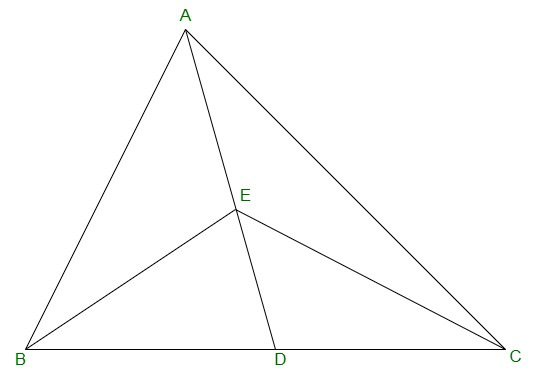Solution:

Given:

Therefore,

It will divide ΔABC into two triangles of equal area.

Therefore,

ar(ABD) = ar(ACD)          -(equation 1)

also,

ED is the median of ΔABC.

Therefore,

ar(EBD) = ar(ECD)          -(equation 2)

Subtracting equation (2) from (1),

ar(ABD) – ar(EBD) = ar(ACD) – ar(ECD)

=> ar(ABE) = ar(ACE)

### Question 2. In a triangle ABC, E is the mid-point of median AD. Show that ar(BED) = 1/4 ar(ABC).

Solution: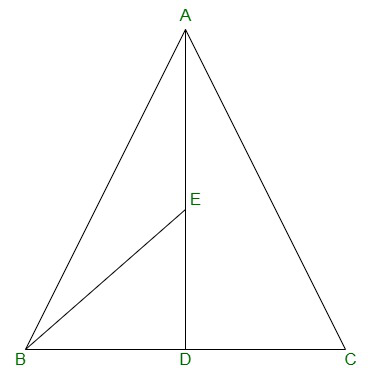ar(BED) = (1/2)×BD×DE

Thus,

E is the mid-point of AD,

AE = DE

Thus,

AD is the median on side BC of triangle ABC,

BD = DC

DE = (1/2) AD          -(equation 1)

BD = (1/2)BC          -(equation-2)

From equation (1) and (2), we get,

ar(BED) = (1/2) × (1/2)BC × (1/2)AD

=> ar(BED) = (1/2) × (1/2)ar(ABC)

=> ar(BED) = 1/4 ar(ABC)

### Question 3. Show that the diagonals of a parallelogram divide it into four triangles of equal area.

Solution: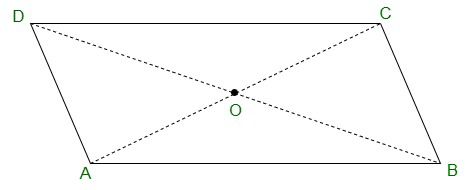O is the mid point of AC and BD. (as diagonals bisect each other)

In ΔABC, BO is the median.

Therefore,

ar(AOB) = ar(BOC)          -(equation 1)

also,

In ΔBCD, CO is the median.

Therefore,

ar(BOC) = ar(COD)          -(equation 2)

In ΔACD, OD is the median.

Therefore,

ar(AOD) = ar(COD)          -(equation 3)

In ΔABD, AO is the median.

Therefore,

ar(AOD) = ar(AOB).         -(equation4)

From equations (1), (2), (3) and (4), we have,

ar(BOC) = ar(COD) = ar(AOD) = ar(AOB)

Therefore,

we get, the diagonals of a parallelogram divide it into four triangles of equal area.

### Question 4. In Figure, ABC and ABD are two triangles on the same base AB. If line- segment CD is bisected by AB at O, show that: ar(ABC) = ar(ABD).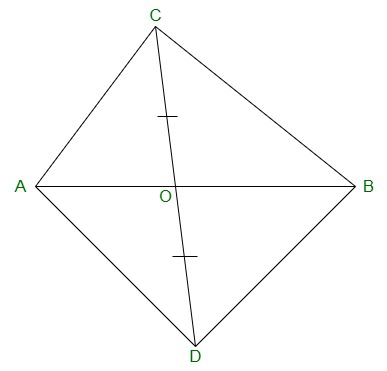Solution:

In ΔABC, AO is the median. (CD is bisected by AB at O)

Therefore,

ar(AOC) = ar(AOD)          -(equation 1)

also,

ΔBCD, BO is the median. (CD is bisected by AB at O)

Therefore,

ar(BOC) = ar(BOD)          -(equation 2)

We will get,

ar(AOC)+ar(BOC) = ar(AOD)+ar(BOD)

=> ar(ABC) = ar(ABD)

### Question 5. D, E and F are respectively the mid-points of the sides BC, CA, and AB of a ΔABC. Show that

(i) BDEF is a parallelogram.

(ii) ar(DEF) = 1/4 ar(ABC)

(iii) ar (BDEF) = 1/2 ar(ABC)

Solution: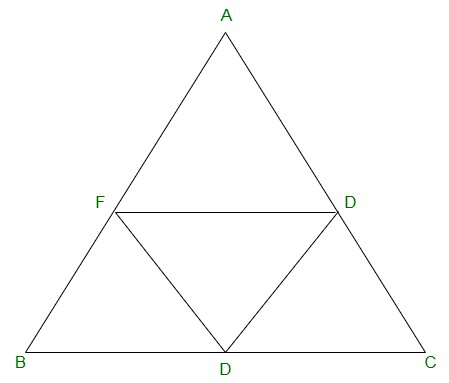(i) In ΔABC,

EF || BC and EF = 1/2 BC (by mid point theorem)

also,

BD = 1/2 BC (D is the mid point)

So,

BD = EF

also,

BF and DE are parallel and equal to each other.

Therefore,

The pair opposite sides are equal in length and parallel to each other.

Hence, BDEF is a parallelogram.

(ii) Proceeding from the result of (i),

BDEF, DCEF, AFDE are parallelograms.

Diagonal of a parallelogram divides it into two triangles of equal area.

Therefore,

ar(ΔBFD) = ar(ΔDEF) (For parallelogram BDEF)          -(equation 1)

also,

ar(ΔAFE) = ar(ΔDEF) (For parallelogram DCEF)          -(equation 2)

ar(ΔCDE) = ar(ΔDEF) (For parallelogram AFDE)          -(equation 3)

From equations (1), (2) and (3)

ar(ΔBFD) = ar(ΔAFE) = ar(ΔCDE) = ar(ΔDEF)

=> ar(ΔBFD) + ar(ΔAFE) + ar(ΔCDE) + ar(ΔDEF) = ar(ΔABC)

=> 4 ar(ΔDEF) = ar(ΔABC)

=> ar(DEF) = 1/4 ar(ABC)

(iii) Area (parallelogram BDEF) = ar(ΔDEF) + ar(ΔBDE)

=> ar(parallelogram BDEF) = ar(ΔDEF) + ar(ΔDEF)

=> ar(parallelogram BDEF) = 2× ar(ΔDEF)

=> ar(parallelogram BDEF) = 2× 1/4 ar(ΔABC)

=> ar(parallelogram BDEF) = 1/2 ar(ΔABC)

### Question 6. In Figure, diagonals AC and BD of quadrilateral ABCD intersect at O such that OB = OD.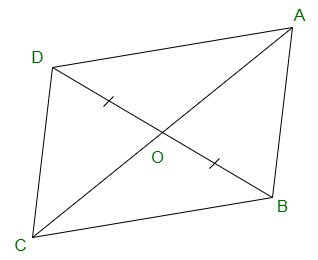If AB = CD, then show that:

(i) ar (DOC) = ar (AOB)

(ii) ar (DCB) = ar (ACB)

(iii) DA || CB or ABCD is a parallelogram.

[Hint : From D and B, draw perpendiculars to AC.]

Solution: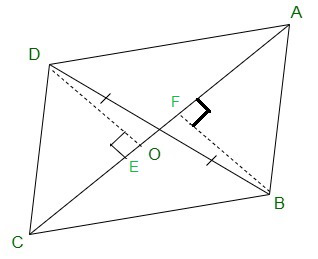Given:

OB = OD and AB = CD

Construct,

DE ⊥ AC and BF ⊥ AC.

To proof:

(i) In ΔDOE and ΔBOF,

∠DEO = ∠BFO (Perpendiculars)

∠DOE = ∠BOF (Vertically opposite angles)

OD = OB (Given)

Therefore,

ΔDOE ≅ ΔBOF by AAS congruence condition.

Therefore, DE = BF (By CPCT)          -(equation 1)

also,

ar(ΔDOE) = ar(ΔBOF) (Congruent triangles)          -(equation 2)

Now,

In ΔDEC and ΔBFA,

∠DEC = ∠BFA (as they are perpendiculars)

CD = AB (Given)

DE = BF (From equation 1)

Therefore,

ΔDEC ≅ ΔBFA by RHS congruence condition.

Therefore,

ar(ΔDEC) = ar(ΔBFA) (Congruent triangles)          -(equation 3)

ar(ΔDOE) + ar(ΔDEC) = ar(ΔBOF) + ar(ΔBFA)

=> ar (DOC) = ar (AOB)

(ii) ar(ΔDOC) = ar(ΔAOB)

Adding ar(ΔOCB) in LHS and RHS,

we will get,

=> ar(ΔDOC) + ar(ΔOCB) = ar(ΔAOB) + ar(ΔOCB)

=> ar(ΔDCB) = ar(ΔACB)

(iii) When two triangles have same base and equal areas,

the triangles will be in between the same parallel lines

ar(ΔDCB) = ar(ΔACB)

DA || BC          -(equation 4)

For quadrilateral ABCD, one pair of opposite sides are

equal (AB = CD) and other pair of opposite sides are parallel.

Therefore,

ABCD is parallelogram.

### Question 7. D and E are points on sides AB and AC, respectively of ΔABC such that ar(DBC) = ar(EBC). Prove that DE || BC.

Solution: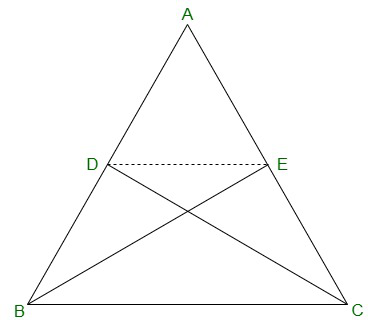ΔDBC and ΔEBC are on the same base BC and are having equal areas.

Therefore,

They will lie between the same parallel lines.

Therefore,

DE || BC.

### Question 8. XY is a line parallel to side BC of a triangle ABC. If BE || AC and CF || AB meet XY at E and F respectively, show that ar(ΔABE) = ar(ΔACF)

Solution: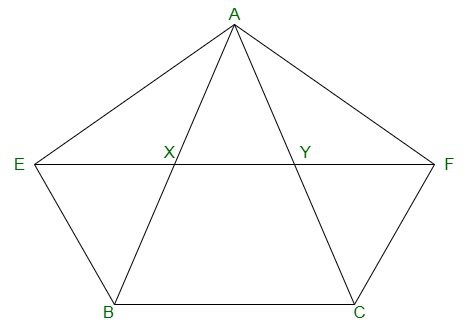Given,

XY || BC, BE || AC and CF || AB

We have to show that,

ar(ΔABE) = ar(ΔAC)

Proof:

BCYE is a parallelogram as ΔABE and ||gm BCYE are on the same

base BE and between the same parallel lines BE and AC.

Therefore,

ar(ABE) =  1/2 ar(BCYE)          -(equation 1)

Now,

CF || AB and XY || BC

=> CF || AB and XF || BC

=> BCFX is a parallelogram.

Thus ΔACF and parallelogram BCFX are on the same base CF

and in-between the same parallel AB and FC. Therefore,

ar (ΔACF)= 1/2 ar (BCFX)          -(equation 2)

But,

Parallelogram BCFX and parallelogram BCYE are on the same

base BC and between the same parallels BC and EF. Therefore,

ar (BCFX) = ar(BCYE)          -(equation 3)

From equations (1), (2) and (3),

We will get,

ar (ΔABE) = ar(ΔACF)

=> ar(BEYC) = ar(BXFC)

As the parallelograms are on the same base BC and

in-between the same parallels EF and BC          -(equation 3)

Also,

ΔAEB and parallelogram BEYC are on the same base

BE and in-between the same parallels BE and AC.

=> ar(ΔAEB) = 1/2 ar(BEYC)          -(equation 4)

Similarly,

ΔACF and parallelogram BXFC on the same base CF

and between the same parallels CF and AB.

=> ar(ΔACF) = 1/2 ar(BXFC)          -(equation 5)

From equations (3), (4) and (5),

ar(ΔABE) = ar(ΔACF).

### Question 9. The side AB of a parallelogram ABCD is produced to any point P. A line through A and parallel to CP meets CB produced at Q and then parallelogram PBQR is completed (see Figure). Show that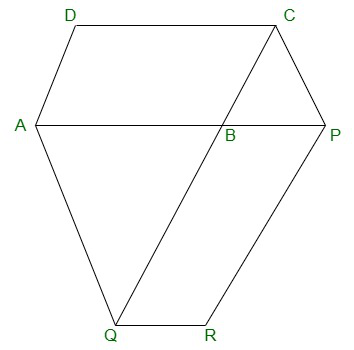### [Hint : Join AC and PQ. Now compare ar(ACQ) and ar(APQ).]

Solution: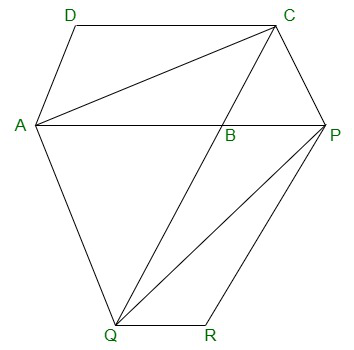AC and PQ are joined.

Ar(ΔACQ) = ar(ΔAPQ)(On the same base AQ and between

the same parallel lines AQ and CP)

=> ar(ΔACQ)-ar(ΔABQ) = ar(ΔAPQ)-ar(ΔABQ)

=> ar(△ABC) = ar(△QBP)          -(equation 1)

AC and QP are diagonals ABCD and PBQR.

Therefore,

ar(ABC) = 1/2ar(ABCD)          -(equation 2)

ar(QBP) = 1/2 ar(PBQR)         -(equation 3)

From equation (2) and (3),

1/2 ar(ABCD) = 1/2 ar(PBQR)

=> ar(ABCD) = ar(PBQR)

### Question 10. Diagonals AC and BD of a trapezium ABCD with AB || DC intersect each other at O. Prove that ar (AOD) = ar (BOC).

Solution: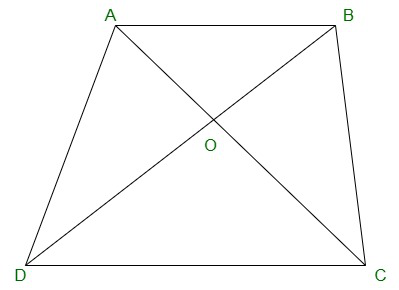ΔDAC and ΔDBC lie on the same base DC and

between the same parallels AB and CD.

Ar(ΔDAC) = ar(ΔDBC)

=>  ar(ΔDAC) – ar(ΔDOC) = ar(ΔDBC) – ar(ΔDOC)

=> ar(ΔAOD) = ar(ΔBOC)

### Chapter 9 Areas of Parallelograms And Triangles – Exercise 9.3 | Set 2

My Personal Notes arrow_drop_up
Related Tutorials